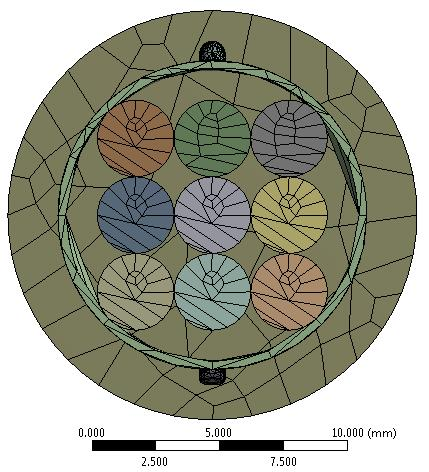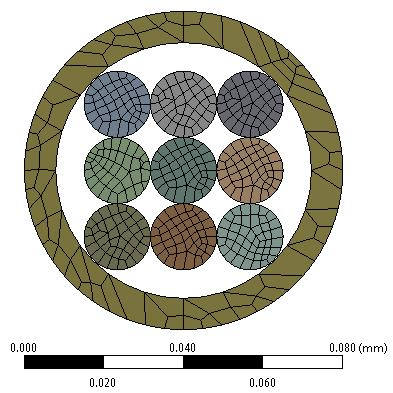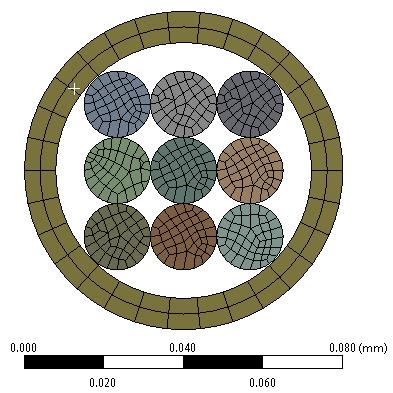## General Mechanical

•masud407
Subscriber

Hello,

I have done a modal analysis for a geometry where dimensions were in millimeters and mode shapes come within 100 Hz. However, I was trying to do modal analysis for a geometry having a very small dimension (in micron). In that case, the modal shape comes at a very high-frequency range ( more than 4000 Hz). Is it okay to find this type of behavior for a geometry with a very size (in microns)? If yes, what can be the reason? How can I solve this issue?

Thanks

•peteroznewman
Subscriber

It is possible to adjust the thickness and length of a cantilever beam and adjust the mass at the end of that beam to get a 100 Hz first natural frequency.

You can do that at the meter scale, the millimeter scale or the micron scale.

I expect the reason you have high frequencies in the micron scale model is because the cantilever beam is too thick as there are manufacturing limitations on how thin layers can be and be made reliably.

•masud407
Subscriber

Hello Peter,

Thanks for your immediate response. I tried with reducing the length. Unfortunately, I found the almost same result. Should I change the thickness? Yes, the thickness was very low (5 microns).

•masud407
Subscriber

I changed the thickness from 5 microns to 15 microns. Still, no change. Mass and volume of the geometry are 1.5* e-9 kg and 1.48*e-3 mm^3 respectively.

•peteroznewman
Subscriber

If you want to lower the first natural frequency of a cantilever beam with a mass at the tip, you must reduce the thickness of the beam, increase the length of the beam or increase the tip mass.

•masud407
Subscriber

Hello Peter,

I changed the thickness and length. Still, the principal natural frequency comes at 200 Hz. I have attached the wbpz file. In A, I used the model where dimensions were in millimeters and natural frequency were below 100 Hz. However, in B, the dimensions were in microns and natural frequencies were more than 200 Hz although the geometry was almost the same. It will be really appreciated if you can have a look at that file.

•peteroznewman
Subscriber

In system A, there is an object that is over 10 mm in diameter. When you were talking about microns, I thought you were talking about MEMS structures.This mesh is of very poor quality and when you run the Modal, a Warning message is issued.Maybe this warning will go away when the mesh is refined. In any case, the natural frequencies are as you found < 100 Hz.
I note that the materials in system A are called membrane, and Muscle as bone.

In system B, you have geometry that is much smaller.You can add a Face Meshing control to the front face to improve the mesh.After I changed the materials to match the first model, the frequencies are much higher than the model at the mm scale.

One important difference, in system A, you are only holding the model at one end, making a cantilever structure, while in system B, you are holding the model at both ends.  Suppressing the fixed support at one end cuts the first natural frequency to be down to 190 Hz.

Another difference is the aspect ratio.

• System A is 43 mm long and a diameter of about 12 mm so a length to diameter ratio of 3.6

• System B is 0.4 mm long and a diameter of about 0.08 mm so a length to diameter ratio of 5

System A is all bonded contact, while system B has some No Separation contact.

Why are you asking about the difference between mm and microns when you have so many other differences?

•masud407
Subscriber

Hello Peter,

I come up with the flaws (geometry ratio, the same type of fixed support, same contact)that you mentioned before. Still, the natural frequency for B is way higher than A.

•peteroznewman
Subscriber

If you have a steel cantilever beam, 100 mm long x 10 mm wide x 1 mm thick it has a natural frequency of 82 Hz.

If you have a steel cantilever beam, 1000 mm long x 100 mm wide x 10 mm thick it has a natural frequency of 8.2 Hz.

So scaling the dimensions up by a factor of 10 results in a change in the first natural frequency by a factor of 1/10.

Why is that? Natural frequency is proportional to the square root of stiffness/mass.

When the cantilever beam is increased in linear dimensions by a factor of 10, the mass goes up by a factor of 1000.

The stiffness of a cantilever beam is proportional to I divided by L^3 where I is the area moment of inertia.

I is proportional to width b times thickness t cubed, therefore the stiffness is proportional to I/L^3= b*t^3/L^3.

If all dimensions increase by a factor of 10, that means I/L^3 increases by a factor of 10.

The square root of stiffness/mass is sqrt(10/1000) = sqrt(1/100) = 1/10.

Therefore we expect the frequency of an object 10 times larger would be 1/10 of the frequency of the small object.